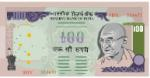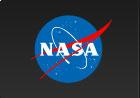# What is Dimensional Analysis?Your grandparents just returned home from a once-in-a-lifetime trip around the world and have offered to give you some of the currency they had left over from the many countries they visited. You can only pick one currency to receive as they are planning to give your siblings the other currencies. You have the following choices:

501.5 Chinese yuan
3288.3 Indian rupees
509.10 Mexican pesos

Of course, you want to choose the one that is worth the most U.S. dollars, but which one would that be? How would you calculate which currency amount is worth the most U.S. dollars?

In order to determine which currency amount is worth the most U.S. dollars, you will first need to know the exchange rate. Currency exchange rates vary daily. For the purpose of this lesson, use the following exchange rates:

1 USD (United States dollar) = 6.5 CNY (Chinese yuan)
1 USD (United States dollar) = 54.8 INR (Indian rupee)
1 USD (United States dollar) = 12.75 MXN (Mexican peso)

Given those exchange rates, can you determine which currency you would want to choose?

In order to determine which one is worth the most U.S. dollars, you will need to convert each to U.S. dollars. You can use dimensional analysis, or factor label method, to convert the currencies.

# Common Unit Conversions in Science

The example in the previous section is a real world application of dimensional analysis. Dimensional analysis is used in science quite often. In this section, you will look at common unit conversions used in science.

Listed below are some other common unit conversions as well as common metric prefixes used in science.

Mass & Weight

1 kg = 1000 g = 2.205 pounds

1 gram = 1000 mg

1 pound = 453.59 grams

1 amu = 1.6606 x 10-24 grams

1 gram = 6.022 x 1023 amu

1 ton = 2000 grams

16 ounces = 1 pound

Length

1 inch = 2.54 cm

1 metere= 100 cm = 39.7 inches

1 yard = 0.9144 meters

1 mile = 1.6093 kilometers

1 kilometer = 1000 meters = 0.621371 miles

1 Angstrom = 1.0 x 10-10 meters = 1.0 x 10-8 cm

1 troy ounce = 31.3 g

Volume

1 liter = 0.001 cubic meters (M3)

1 liter = 1000 cm3 = 1000 mL

1 liter = 1.056 quarts

1 quart = 0.94631 liter

1 mL = 0.001 liters = 1 cm3

1 ft3 = 7.475 gallons = 28.316 liters

1 cup = 16 ounces

1 quart = 4 cups = 2 pints

4 quarts = 1 gallon

1 ounce = 29.57 cm3 or mL

Metric Conversions
Unit Abbr.

Meaning (Example: 1 gram = 103 kg)

Mega- M 106

kilo-

k 103
hecto- h 102
deca- dk 101
deci- d 10-1
centi- c 10-2
milli- m 10-3
micro- µ 10-6
nano- n 10-9

Let’s practice a few unit conversions using dimensional analysis. Remember the steps we learned in section 1.

1. Write the given information as a fraction by placing it over 1. (Placing it over 1 makes it a fraction but does not change its value.)
2. Write a conversion factor that has the unit you want to remove in the denominator and the unit you want to end up with in the numerator. After you fill in your units, add the numbers. (Usually one of the numbers is a 1, but it can be in either the denominator or the numerator.) Note: In some cases you may need to repeat this step a number of times in order to get the unit you want to end up with in the numerator.
3. Mark through the units to double check that they all cancel and that you are left with the units you want.
4. Multiply the numbers in the numerators, and then multiply the numbers in the denominators.

Try these problems

# Dimensional Analysis Practice 1Let’s practice more problems using dimensional analysis. The following two problems are real world famous unit conversion errors from Space Math @ NASA.

Directions: Use dimensional analysis to see if you can answer the problems.

Problem 1
Background: On September 23, 1999, NASA lost the \$125 million Mars Climate Orbiter spacecraft after a 286-day journey to Mars. Miscalculations, using English units instead of metric units, slowly sent the craft 60 miles off course. Lockheed Martin, the aerospace company performing the calculations, was sending thruster data in English units (pounds) to NASA, while NASA's navigation team was expecting metric units (newtons). Thrusters used to help point the spacecraft had, over the course of months, been fired incorrectly because the data used to control the wheels were calculated in incorrect units.

Problem 2
Background: On January 26, 2004, Tokyo's Disneyland Space Mountain broke an axle on a roller coaster train mid-ride causing it to derail. It was found that the wrong size part was used due to a conversion miscalculation of the master plans in 1995 from English units to metric units. In 2002, new axles were mistakenly ordered using the pre-1995 English specifications instead of the current metric specifications.

# Dimensional Analysis Practice 2

The following problems are a little bit harder. Be sure and read each question carefully. If you need a hint, click on the hint button.